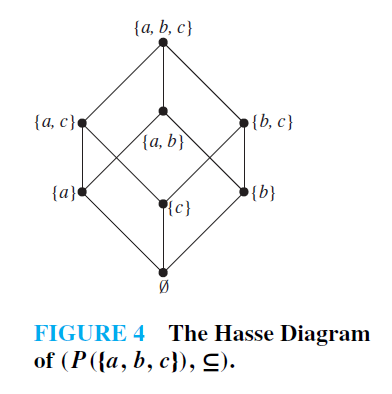An order is a binary relation  on a set , written .

• If two things and are incomparable, we write it
• Total order:  for all a and b in the set, either  or
• Partial order: at least one pair a and b in the set, where  or

## Joins §

An upper bound is an element of the set that is every other element in the set in terms of that relation

When we take the join of and (written ), we’re looking for some element for which and where is the smallest element that satisfies that condition

Join has

1. Commutativity:
2. Associativity:
3. Idempotence:

When it comes to CRDTs, what we’re looking for is the ability to apply an operation in any order and as many times as we want without corrupting the result. The laws obeyed by joins give us exactly this.

A join semi-lattice then essentially does a topological sort or causal ordering of its elements except all of the elements can be joined (i.e. have a single shared ancestor)

We can illustrate the semi-lattice using a Hasse Diagram